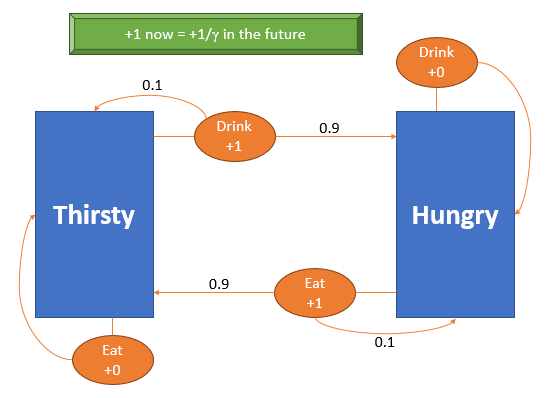## Posts

Showing posts from September, 2018

### Markov Decision Processes 02: how the discount factor worksPt En < change language In this previous post I defined a Markov Decision Process and explained all of its components; now, we will be exploring what the discount factor $\gamma$ really is and how it influences the MDP. Let us start with the complete example of last post: In this MDP the states are Hungry and Thirsty (which we will represent with $H$ and $T$) and the actions are Eat and Drink (which we will represent with $E$ and $D$). The transition probabilities are specified by the numbers on top of the arrows. In the previous post we put forward that the best policy for this MDP was defined as $$\begin{cases} \pi(H) = E\\ \pi(T) = D\end{cases}$$ but I didn't really prove that. I will do that in a second, but first what are all the other possible policies? Well, recall that the policy $\pi$ is the "best strategy" to be followed, and $\pi$ is formally seen as a function from the states to the actions, i.e. $\pi: S \to A$. Because of that, we m

### Markov Decision Processes 01: the basicsPt En < change language In this post I will introduce Markov Decision Processes, a common tool used in Reinforcement Learning, a branch of Machine Learning. By the end of the post you will be able to make some sense of the figure above! I will couple the formal details, definitions and maths with an intuitive example that will accompany us throughout this post. In later posts we will make our example more complete and use other examples to explain other properties and characteristics of the MDPs. Let me introduce the context of the example: From a simplistic point of view, I only have two moods: " hungry " and " thirsty ". Thankfully, my parents taught me how to eat and how to drink, so that I can fulfill the needs I mentioned earlier. Of course that eating when I am hungry makes me happy, just as drinking when I am thirsty makes me happy! Not only that, but eating when I am hungry usually satisfies me, much like drinking when I am thirsty us

### Twitter proof: folding my way to the moonPt En < change language In this twitter proof we will see how the exponential function can mess up with objects from our daily lives!.. Claim: with less than $50$ folds, a piece of paper will be so thick that it will cover the distance from the Earth to the Moon. Twitter proof: a common sheet of paper is $0.1$mm thick. If we fold it once, it becomes $0.2$mm thick. Folding twice, $0.4$mm. Folding $49$ times, the paper becomes $2^{49}\times 0.1$mm thick, which is around $5.63\times 10^{13}$mm or $5.63\times10^7$km, $141$ times the distance from the Earth to the Moon ($398818$km). Neste post vamos ver como a função exponencial pode interagir com objetos do nosso quotidiano e criar resultados inesperados. Proposição: com menos de $50$ dobras, uma folha de papel fica com uma grossura superior à distância da Terra à Lua. Prova num tweet: uma folha de papel normal tem $0.1$mm de grossura. Se a dobrarmos uma vez, fica com $0.2$mm de grossura. Dobrando de nov